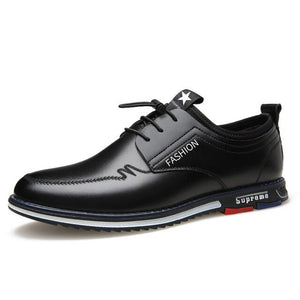# Men New Fashion Flat Luxury Italian Shoes

• \$50.99 USD
単価 あたり
• 以下の金額をセーブしました。 \$45.00 USD

US6.0=EU38=240mm=9.45"(bare feet length)
US6.5=EU39=245mm=9.65"
US7.0=EU40=250mm=9.84"
US8.0=EU41=255mm=10.04"
US8.5=EU42=260mm=10.24"
US9.5=EU43=265mm=10.43"
US10.0=EU44=270mm=10.63"
US11.0=EU45=275mm=10.83"
US12.0=EU46=280mm=11.02"
US12.5=EU47=285mm=11.2

US13.0=EU48=290mm=11.42"

Specification:
Sole Material: Rubber
Function: breathable
Applicant: youth (18-40 years old)